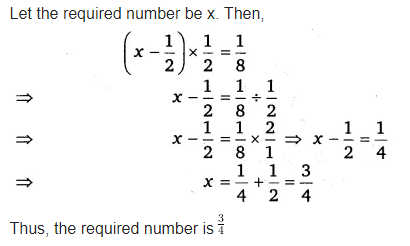# If you subtract 1/2 from a number and multiply the result 1/2 by you get 1/2 What is the number?

If you subtract 1/2 from a number and multiply the result 1/2 by you get 1/8 What is the number ?